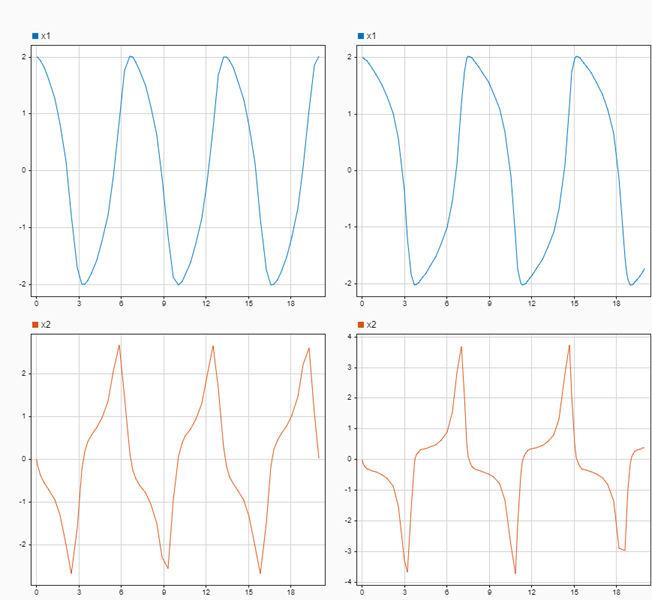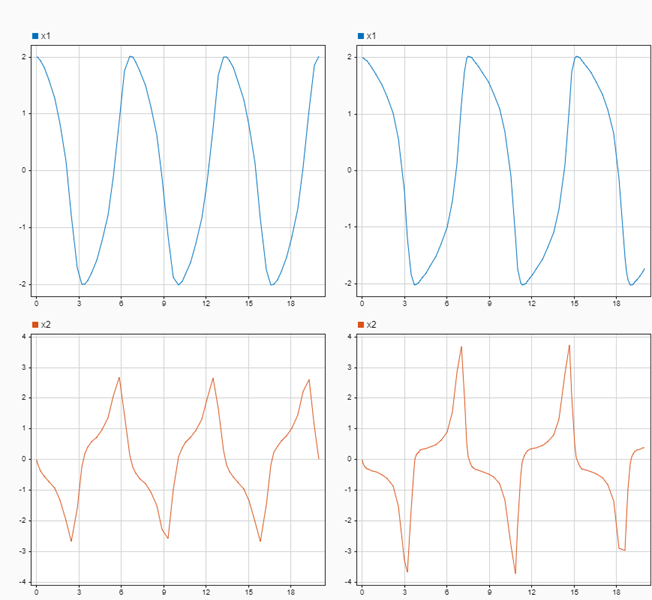Get t- and y-axis limits for time plot in the Simulation Data Inspector

## Syntax

``[tMin,tMax,yMin,yMax] = Simulink.sdi.getSubplotLimits(r,c)``

## Description

example

````[tMin,tMax,yMin,yMax] = Simulink.sdi.getSubplotLimits(r,c)` returns the t- and y-axis limits for the subplot at the specified location in the Simulation Data Inspector subplot layout.```

## Examples

collapse all

You can use the `Simulink.sdi.getSubplotLimits` and `Simulink.sdi.setSubplotLimits` functions to copy the axis limits from one subplot to another. For example, you can specify the same y-axis limits for two subplots that display the same signal from simulations that used different values of a model parameter. This example copies the y-axis settings from one subplot to another to analyze the effect of changing the value of `Mu` in the model `vdp`.

Create the data in the Simulation Data Inspector by simulating the model `vdp` twice. The first time, specify the value of `Mu` as `1`. For the second simulation, set the value of `Mu` to `2`. The model logs data for the signals `x1` and `x2`.

```open_system('ex_vdp'); set_param('ex_vdp/Mu','Gain','1') sim('ex_vdp'); set_param('ex_vdp/Mu','Gain','2') sim('ex_vdp');```

Open the Simulation Data Inspector.

`Simulink.sdi.view`

Create plots in the Simulation Data Inspector to show the results from each simulation. Configure a `2x2` subplot layout so you can plot one signal on each plot, side by side.

`Simulink.sdi.setSubPlotLayout(2,2)`

Get the `Simulink.sdi.Signal` objects that correspond to each signal from each run, and plot one signal on each subplot. Plot the signals from the first simulation on the subplots in the first column and the signals from the second simulation on the subplots in the second column.

```runIDs = Simulink.sdi.getAllRunIDs; runID1 = runIDs(end-1); runID2 = runIDs(end); runMu1 = Simulink.sdi.getRun(runID1); runMu2 = Simulink.sdi.getRun(runID2); sig1 = getSignalByIndex(runMu1,1); sig2 = getSignalByIndex(runMu1,2); sig3 = getSignalByIndex(runMu2,1); sig4 = getSignalByIndex(runMu2,2); plotOnSubPlot(sig1,1,1,true); plotOnSubPlot(sig2,2,1,true); plotOnSubPlot(sig3,1,2,true); plotOnSubPlot(sig4,2,2,true);```

The signals in the first column of plots look similar to those in the second column. However, the y-axis limits for the plots of the `x2` signal are different.Use the `Simulink.sdi.getSubplotLimits` function to copy the limits used for the subplot with the `x2` signal from the second simulation.

`[~,~,ymin,ymax] = Simulink.sdi.getSubplotLimits(2,2);`

Use the `Simulink.sdi.setSubplotLimits` function to apply the same y-axis limits to the subplot with the `x2` signal from the first run.

`Simulink.sdi.setSubplotLimits(2,1,'yRange',[ymin,ymax]);`

With the updated y-axis limits, the difference in the `x2` signal is more apparent.## Input Arguments

collapse all

Subplot row index, specified as an integer between `1` and `8`, inclusive. Use the `r` and `c` inputs together to specify the location of the subplot for which you want the axis limits.

Example: `[~,~,yMin,yMax] = Simulink.sdi.setSubplotLimits(2,2)` returns y-axis limits for the time plot in the second row of the second column of the subplot layout in the Simulation Data Inspector.

Subplot column index, specified as an integer value between `1` and `8`, inclusive. Use the `r` and `c` inputs together to specify the location of the subplot for which you want the axis limits.

Example: `[~,~,yMin,yMax] = Simulink.sdi.setSubplotLimits(2,2)` returns the y-axis limits for the time plot in the second row of the second column of the subplot layout in the Simulation Data Inspector.

## Output Arguments

collapse all

t-axis minimum, returned as a double and the first element in a `1`-by-`4` vector of the form [`tMin,tMax,yMin,yMax`].

t-axis maximum, returned as a double and the second element in a `1`-by-`4` vector of the form [`tMin,tMax,yMin,yMax`].

y-axis minimum, returned as a double and the third element in a `1`-by-`4` vector of the form [`tMin,tMax,yMin,yMax`].

y-axis maximum, returned as a double and the last element in a `1`-by-`4` vector of the form [`tMin,tMax,yMin,yMax`].

## Version History

Introduced in R2021a